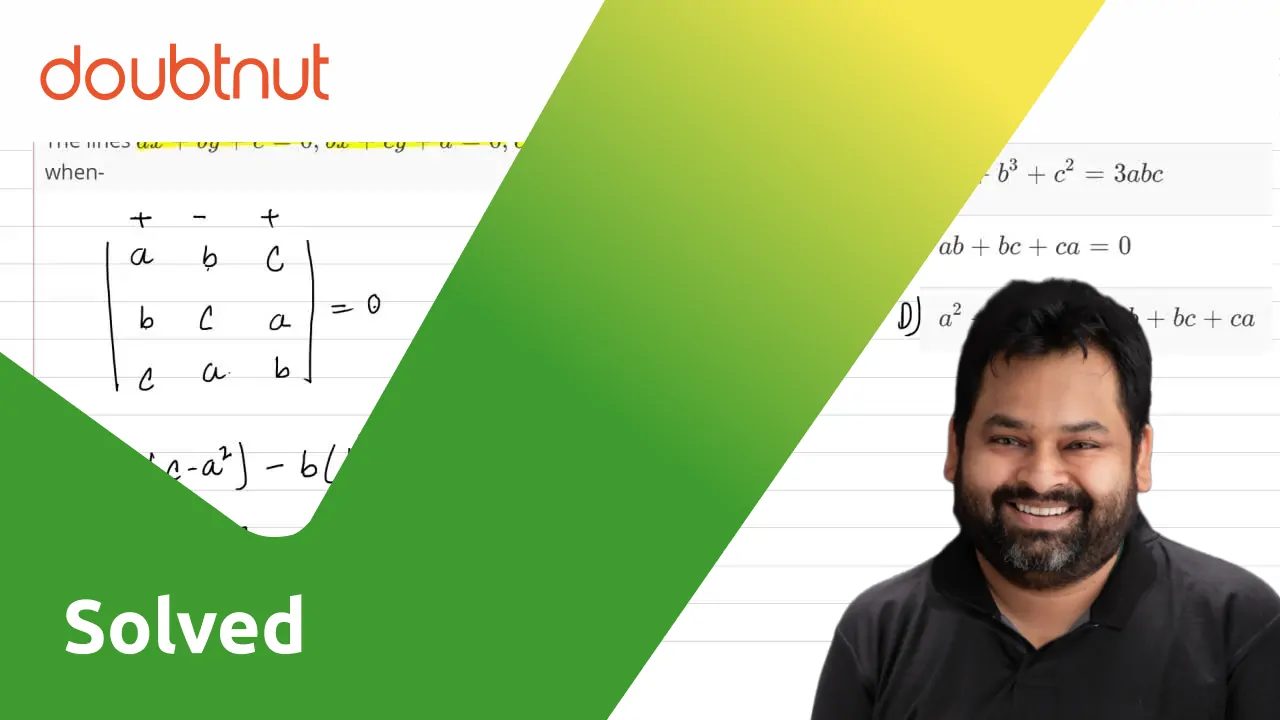Doubtnut App - Get 50 Lakh+ video solutions better than GoogleInstall Now

# The lines ax+by+c=0,bx+cy+a=0,cx+ay+b=0 are concurrent when- Solution in Bengali

A

ab2+bc2+ca2=abc

B

a3+b3+c2=3abc

C

ab+bc+ca=0

D

a2+b2+c2=ab+bc+ca

Text Solution
Verified by Experts

Video Solution